2-minute Get-Started exercises Download and install Basic input/output Sample Mathematics Vectors and vector commands Matrices and matrix commands Solve linear algebraic equations Solve nonlinear algebraic equations Solve ODEs (differential equations)
 Additional exercises Units and conversion factors Default preferences Plotting data and functions

 MotionGenesis Kane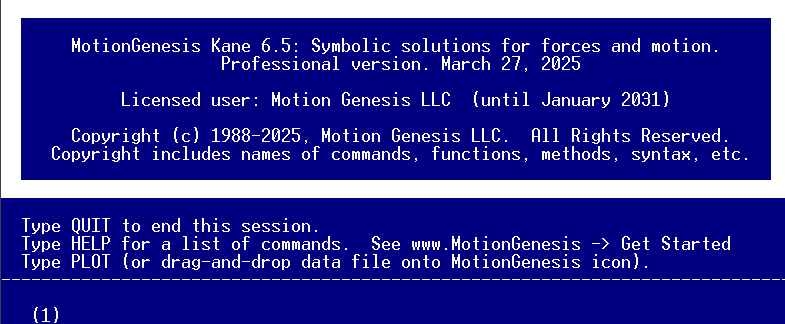Get started: ShortAndFast  .pdf Details: MotionGenesisTutorial.pdf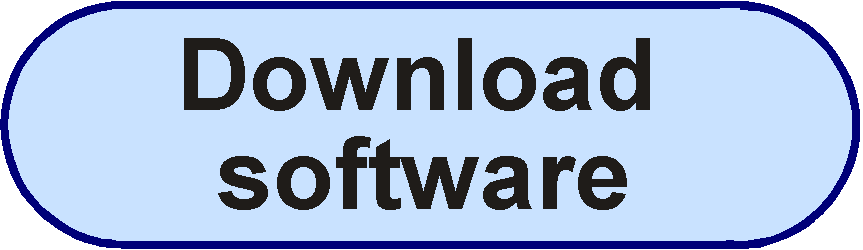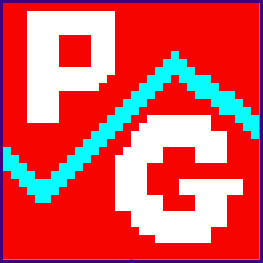PlotGenesis

Sample problems

1D motion   Projectiles(4) -- with air-resistance     Single pendulum(10)     Double(3)     Air/spacecraft spin stability     Angular momentum and gyros(4)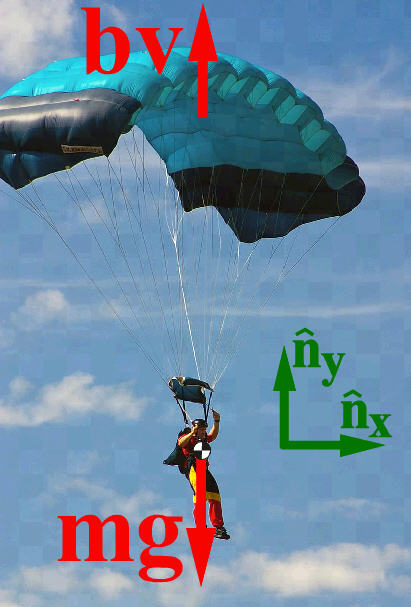Event handling in MATLAB®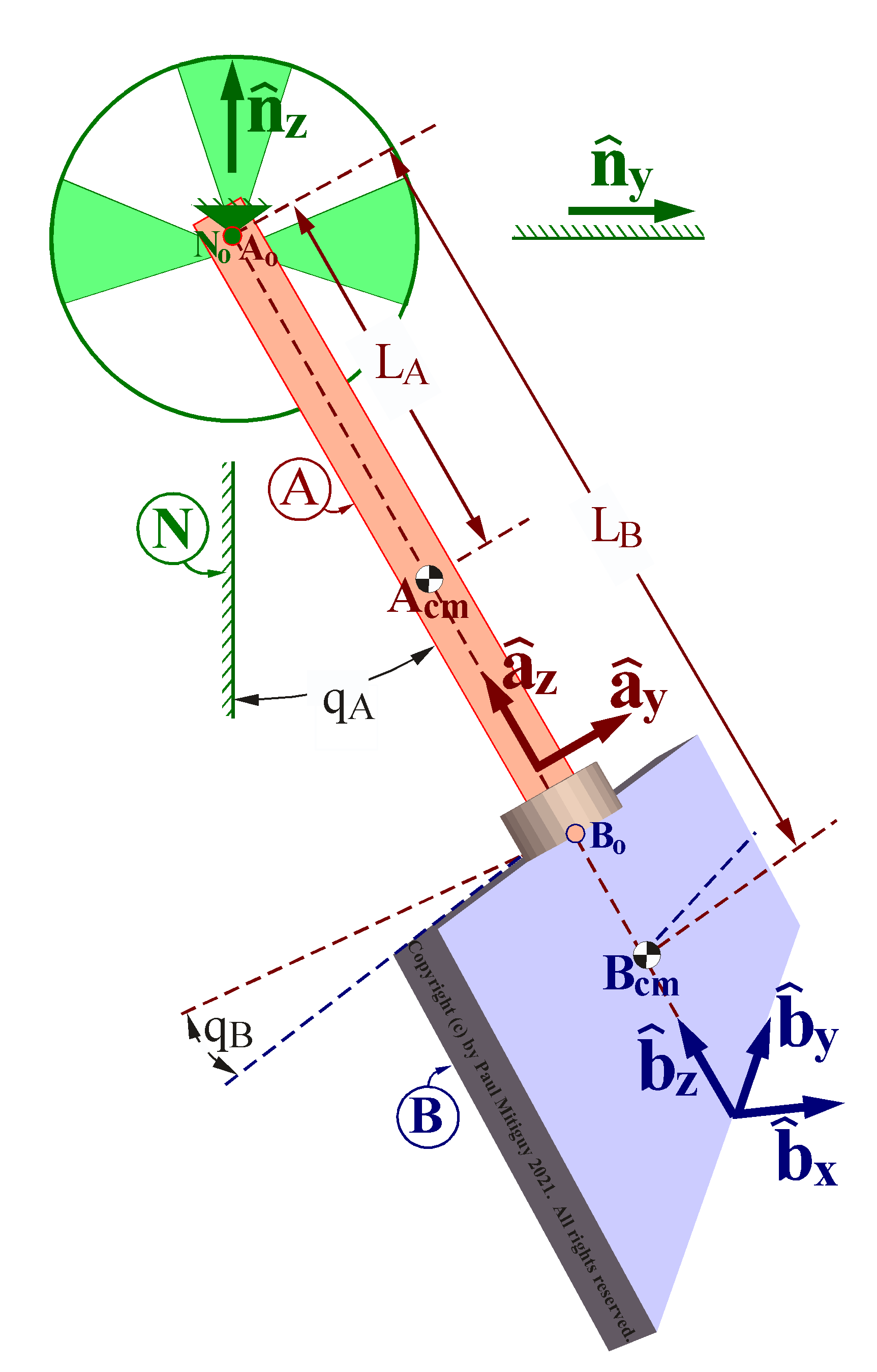Stick/slip(2) 2D/3D Geometry(16) Linkages/mechanisms Rolling disks, unicycle, ... One-wheel trailer Rattleback (Dynamic Celt)Statics(5) Simple control Vehicle skid Tricycle stability/control HexapodRobotics(4) Suspension bridge Biomechanics DC motor (resistance & inductance) Mass/inertia